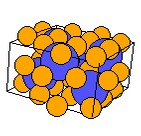Crystal Lattice Structures: Creation Date: 24 Sep 2002 Last Modified: 10 June 2005

# The Bainite (Fe3C) StructureYou can now

• Pearson's Handbook actually lists this as upper Bainite.

• Prototype: Fe3C (Bainite)
• Pearson Symbol: hP8
• Space Group: P6322 (Cartesian and lattice coordinate listings available)
• Number: 182
• Reference: Villars and Calvert, Pearson's Handbook Vol. II, p. 1894.
• Primitive Vectors:  A1 = ½ a X - ½ 3½ a Y A2 = ½ a X + ½ 3½ a Y A3 = c Z
• Basis Vectors:  B1 = 1/3 A1 + 2/3 A2 + 3/4 A3 = ½ a X  + 12-½ a Y  + 3/4 c Z (2d) (C) B2 = 2/3 A1 + 1/3 A2 + 1/4 A3 = ½ a X  - 12-½ a Y  + 1/4 c Z (2d) (C) B3 = x A1 = ½ x a X  - ½ 3½ x a Y (6g) (Fe) B4 = x A2 = ½ x a X  + ½ 3½ x a Y (6g) (Fe) B5 = - x A1 - x A2 = - x a X (6g) (Fe) B6 = - x A1 + 1/2 A3 = - ½ x a X  + ½ 3½ x a Y  + ½ c Z (6g) (Fe) B7 = - x A2 + 1/2 A3 = - ½ x a X  - ½ 3½ + x a Y  + ½ c Z (6g) (Fe) B8 = x A1 + x A2 + ½ c Z = x a X  + ½ c Z (6g) (Fe)

Go back to the hexagonal close-packed and related structures page.

Go back to Crystal Lattice Structure page.

 Structures indexed by: This is a mirror of an old page created at theNaval Research LaboratoryCenter for Computational Materials ScienceThe maintained successor is hosted at http://www.aflowlib.org/CrystalDatabase/ and published as M. Mehl et al., Comput. Mater. Sci. 136 (Supp.), S1-S828 (2017).